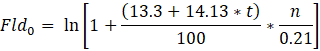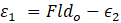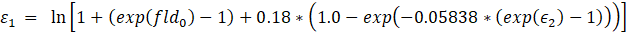# How Is the Forming Limit Curve Calculated?

## Question

How is the Forming Limit Curve calculated?

## Remedy

FLC in true strain space is calculated by the following equations

Fldo calculated byt = Thickness (mm)

n = strain hardening

Left side of the curve is calculated byRight side of the curve is calculated by